Next: Output and Interaction Issues Up: Stochasticks: Augmenting the Previous: Color Model Classification

# Strategy and Planning

We now describe a strategy for selecting the shot that is most likely to succeed. Starting from the cue-ball, we compute linear trajectories to all solid balls (or striped balls if the user selects to play as stripes). We then compute linear trajectories from these to the detected pockets. Then we test each of these possible trajectories: from cue-ball to solid-ball to pocket to find those that don't involve any collisions with other objects. If no solid balls are found, we test the 8-ball for possible trajectories.

We then weight each of the 'valid' trajectories by the following heuristics. Each time the user is to take a shot, we are really interested in the angle at which he or she hits the cue ball (we shall assume that the force with which the cue ball is hit is going to be determined by the user). So, what is fundamentally being suggested to the user is an angle at which to hit a cue ball, say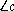as a deviation from the line connecting the cue ball to the solid ball. The more accuracy we need on, the harder the shot. Figureshows how we can determine the required angle. The distance from the cue-ball to the solid-ball is l and the distance from there to the pocket is L. The angle formed by the three objects is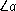at the solid ball. We can computeusing this information as well as a bound on the maximum error onwhich is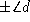.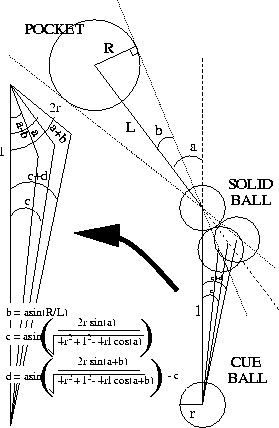Figure: Determining the Angle and the Difficulty

For each possible shot, we compute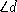and display to the user the shot with the greatest value of(the shot which requires the least accuracy) with the required trajectory (along) superimposed on the image. This is a simplified first-order strategy model since it is only 2D and does not include spin effects, kinematics or rebounds in the computation of the shots.Next: Output and Interaction Issues Up: Stochasticks: Augmenting the Previous: Color Model Classification

Tony Jebara
Wed Feb 18 18:52:15 EST 1998